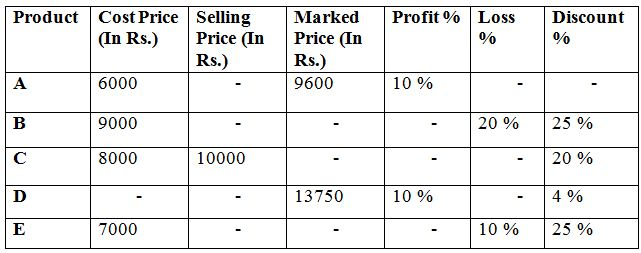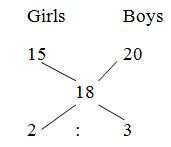# NIACL AO Prelims – Quantitative Aptitude Questions Day- 78

Dear Readers, Bank Exam Race for the Year 2019 is already started, To enrich your preparation here we have providing new series of Practice Questions on Quantitative Aptitude – Section. Candidates those who are preparing for NIACL AO Prelims 2019 Exams can practice these questions daily and make your preparation effective.

[WpProQuiz 4909]

1) Naveena’s present age is four times her son’s present age and two fifth of her father’s present age. The average present age of all of them is 40 years. Find the sum of Naveena’s son’s present age and Naveena’s father’s present age?

a) 92 years

b) 74 years

c) 88 years

d) 86 years

e) None of these

2) The average age of girls in the class is 15 years. The average age of boys in the class also added, then the average becomes 18. If there are 18 boys in the class and the average age of boys in the class is 20, then find how many girls in the class?

a) 18

b) 24

c) 12

d) 16

e) None of these

3) A boat can cover 42 km upstream in 63 minutes. If the speed of the current is 3/7 of the boat in still water, then how much distance (in km) can the boat cover downstream in 42 minutes?

a) 70 km

b) 66 km

c) 52 km

d) 84 km

e) None of these

4) P, Q, R started a business with investments of Rs. 12000, Rs. 15000 and Rs. 18000 respectively. After 8 months from the start of the business, Q and R invested additional amounts in the ratio of 3 : 5 respectively. If at the end of the year, the ratio of share of P and Q was 3 : 4, then what was the additional amount invested by Q after 8 months?

a) Rs. 1500

b) Rs. 1800

c) Rs. 2100

d) Rs. 3000

e) None of these

5) Three pipes A, B and C can fill a tank in 10 hours. After working at it together for 3 hours, C is closed and A and B can fill the remaining part in 14 hours. How much time taken by C to fill the tank alone?

a) 18 hours

b) 20 hours

c) 24 hours

d) 22 hours

e) None of these

Directions (Q. 6 – 10):  Study the following information carefully and answer the given questions:

The following table shows the cost price, selling price, marked price, profit and loss % and discount % for the different products.6) If the cost price of product S is 20% more than the cost price of product D. The cost price, selling price, and marked price of product S is in the ratio of 12 : 13 : 15 respectively. Then find the difference between the selling price of product S and the selling price of product C?

a) Rs. 5900

b) Rs. 5600

c) Rs. 6500

d) Rs. 6000

e) None of these

7) Cost price of product F is 10% more than the selling price of product A. Then find the difference between the cost price product F and selling price of product E?

a) Rs. 960

b) Rs. 850

c) Rs. 1080

d) Rs. 1000

e) Rs. 800

8) Average cost price of product A, B and D is Rs. 8000. Then find the ratio between the selling prices of product D to that of the marked price of product E?

a) 14 : 25

b) 19 : 33

c) 21 : 28

d) 33 : 28

e) None of these

9) Cost price of product X is 4/5th of the selling price of product D. The cost price of product Y is 7/8th of marked price of product A. Then cost price of product X is approximately how much percentage more/less than by the cost price of product Y?

a) 35 % more

b) 25 % more

c) 25 % less

d) 40 % more

e) 35 % less

10) Find the difference between the average cost price and average marked price of all the products?

a) Rs. 4760

b) Rs. 4580

c) Rs. 2830

d) Rs. 3520

e) Rs. 2370

The ratio of present age of Naveena and her son’s age = 4 : 1 (4x, x)

Naveena’s present age = (2/5)*Naveena’s father’s present age

Naveena’s father’s present age = 10x

According to the question,

x + 4x + 10x = 120

15x = 120

x = 120/15

x = 8

Naveena’s father’s present age = 10x = 80 years

Naveena’s son’s present age = x = 8 years

Required sum = 80 + 8 = 88 years

Let the total number of girls in the class be x,

15x + (20*18) = 18*(18 + x)

15x + 360 = 324 + 18x

3x = 36

X = 12

Total girls in the class is 12

Shortcuts:3’s = 18

1’s = 6

Total number of girls in the class = 2’s = 12

Speed of upstream = (48*60)/63 = 40 km/hr

Speed of current : Speed of still water = 3 : 7

Speed of upstream = Speed of boat in still water – speed of Current

40 = 4x

= > x = 10

Speed of current = 30 km/hr

Speed of boat in still water = 70 km/hr

Speed of downstream= Speed of boat in still water + speed of Current

= > 70 + 30 = 100 km/hr

Speed = 100 km/hr, Time = 42 minutes

Distance = 100*(42/60) = 70 km

Let the ratio additional amount of Q and R be 3x and 5x,

The ratio of profit of A: B: C

= [12000*12] : [15000*8 + (15000 + 3x) *4] : [18000*8 + (18000 + 5x) *4]

= 144000 : (120000 + 60000 +12x) : (144000+ 72000 + 20x)

The ratio of share of P and Q = 3 : 4

144000/(180000+ 12x) = (3/4)

192000 = 180000 + 12x

12000 = 12x

x = 1000

Q’s additional investment after 8 months = 3x = 3*1000 = Rs. 3000

Three pipes A, B and C can fill a tank in 8 hours.

A, B and C’s 1 hour work=1/10

A, B and C’s 3 hour work= 3/10

Remaining work= 1 – (3/10) = 7/10

The remaining part will be filled by A and B in 6 hours. Then,

= > (7/10) *(A+B) = 14

= > (A+B)’s whole work= 14*(10/7) = 20 hr

(A+B)’s 1 hour work= 1/20

A, B and C’s 1 hour work = 1/10

C’s 1 hour work= (A+B+C) – (A+B)

= > (1/10) – (1/20)

= > 1/20

C can fill the tank in 20 hours.

C.P of product D,

= > 13750*(96/100) = CP*(110/100)

= > CP = Rs. 12000

C.P of product S = 12000 + 2400 = Rs. 14400

SP of product S =14400*(13/12) = Rs. 15600

Required difference = 15600 – 10000 = Rs. 5600

S.P of A= Rs. 6600

C.P of F = 6600*(110/100) = Rs. 7260

Required difference = 7260 – 6300 = Rs. 960

Cost price of A, B and D = 8000*3 = Rs. 24000

Cost price of D = 24000 – 15000 = Rs. 9000

Selling price of D = 9000*(110/100) = Rs. 9900

Marked price of E = 7000*(90/100)*(100/75) = Rs. 8400

Required ratio = 9900 : 8400 = 33 : 28

C. P of X = 13750*(96/100)*(4/5) = Rs. 10560

C.P of Y = 9600*(7/8) = Rs. 8400

Required % = [(10560 – 8400)/8400]*100 = 25 % more

Cost price of product D

= > 13750*(96/100)*(100/110) = Rs. 12000

Average CP = (6000 + 9000 + 8000 + 12000 + 7000)/5 = Rs. 8400

Marked price of product B = 9000*(80/100)*(100/75) = Rs. 9600

Marked price of product C = 10000*(100/80) = Rs. 12500

Marked price of product E = 7000*(90/100)*(100/75) = Rs. 8400

Average MP = (9600 + 9600 + 12500 + 13750 + 8400)/5 = Rs. 10770

Required difference = 10770 – 8400 = Rs. 2370